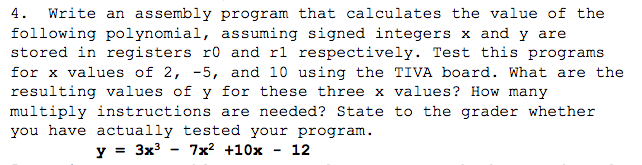# Question & Answer: Write an assembly program that calculates the value of the following polynomial assuming signed…..

Write an assembly program that calculates the value of the following polynomial assuming signed integers x and y are stored in registers r0 and r1 respectively. Test this programs for x values of 2, -5, and 10 using the TIVA board. What are the resulting values of y for these three x values? How many multiply instructions are needed? State to the grader whether you have actually tested your program. Y = 3x^3 – 7x^2 +10x – 12Don't use plagiarized sources. Get Your Custom Essay on
Question & Answer: Write an assembly program that calculates the value of the following polynomial assuming signed…..
GET AN ESSAY WRITTEN FOR YOU FROM AS LOW AS \$13/PAGE

main:

push {r7}
sub sp, sp, #12
ldr r3, [r7, #4]
ldr r2, [r7, #4]
mul r2, r2, r3
mov r3, r2
lsl r3, r3, #1
ldr r2, [r7, #4]
mul r2, r2, r3
ldr r3, [r7, #4]
ldr r1, [r7, #4]
mul r3, r1, r3
mov r1, r3
lsl r3, r3, #3
subs r3, r1, r3
ldr r2, [r7, #4]
mov r3, r2
lsl r3, r3, #2